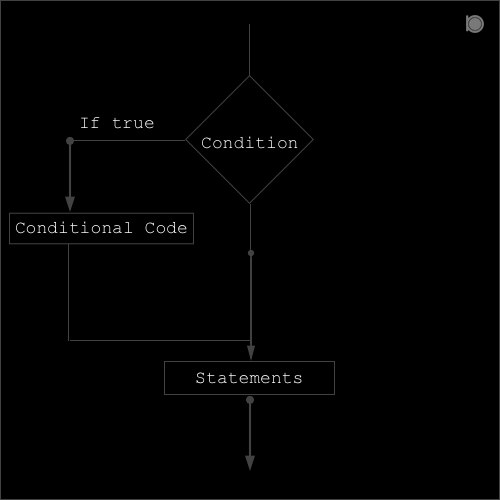if statement in C language contains only true block which means if the specified condition is true then the true block is executed.##### Syntax of if statement:
``````if (condition or expression)
{
conditional code;
}
``````

### Program example of if statement in C

``````//An example of if statement in C
#include<stdio.h>

int main()
{
int a = 10, b = 20;
printf("Consider a = 10 and b = 20\n");
if(a>b)
{
printf("a is greater\n");
}

if(b>a)
{
printf("b is greater\n");
}

printf("This is an example of if statement in C\n");
return 0;
}
``````

### Output

``````Consider a = 10 and b = 20
b is greater
This is an example of if statement in C
``````

In the above example we have two integer variables `a` and `b` having value `10` and `20` respectively. In the first if statement we have a condition `a>b`, since a is not greater b so this block is skipped. And in second if statement we have a condition `b>a` since this condition is true so the conditional code inside the block is executed.